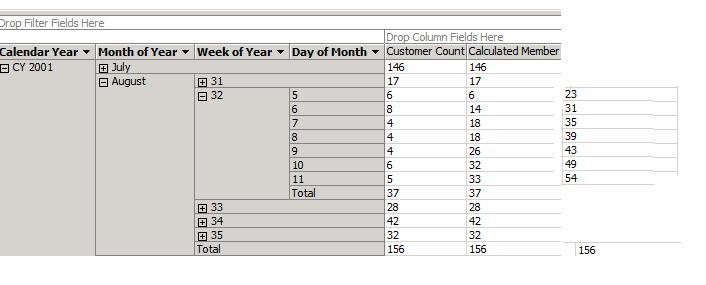#weekly & monthly moving sum• ### Question

•Hi All,

Could you tell me if there is the way to calculate weekly and monthly moving sum the same time. It should looks like this picture

If you open any week : week column  should sum up customer count  by week, though month column should continue calculate from month  beggingActually I have tried to use (adWorksDW)

sum([Date].[Day of Month].currentmember.lag(30):[Date].[Day of Month].currentmember,[Measures].[Customer Count])
and
sum([Date].[Day of Month].currentmember.lag(7):[Date].[Day of Month].currentmember,[Measures].[Customer Count])

Saturday, May 23, 2009 10:45 PM

•Hi Akalya,

Thanks for your help:
month sum:

iif

([Date].[Hierarchy 1].level is [Date].[Hierarchy 1].[Week of Year],null,SUM(periodstodate([Date].[Hierarchy 1].[Month of Year],[Date].[Hierarchy 1].currentmember),[Measures].[Customer Count]))

week sum:

SUM(periodstodate([Date].[Hierarchy 1].[Week of Year],[Date].[Hierarchy 1].currentmember),[Measures].[Customer Count])

Thanks again

• Marked as answer by Tuesday, May 26, 2009 3:04 PM
Tuesday, May 26, 2009 2:05 PM

### All replies

•At a guess I would say that this is not working because you are using the [Day of Month] attribute which probably only has 31 members, therefore if you go .Lag(30) from day 5 it does not work. Try using an attribute based on the date that has a unique member for each date.
Monday, May 25, 2009 7:06 AM
•Hi Darren,

sum([Date].[Date].currentmember.lag(31): [Date].[Date].currentmember,[Measures].[Customer Count])

it works correctly for mothly culculation only, if I use calendarYear->MonthOfYear->WeekOfYear-> Date
-------------------------------------------------------------------------------------------------------------------------------
For week it will work if I chose calendarYear->MonthOfYear->WeekOfYear-> DayOfMonth

sum
( [Date].[Day of Month].currentmember.lag(7) : [Date].[Day of Month].currentmember,[Measures].[Customer Count])

---------------------------------------------

I wonder if you have an idea how combine them

Monday, May 25, 2009 7:48 PM
•I'm not sure what you mean by wanting to combine them. If you want create a pivot table like the image in your first post you would create these as 2 separate measures "31 Day Moving Sum" and "7 Day Moving Sum".
Tuesday, May 26, 2009 3:39 AM
•I am not clear as to why is there such a requirement. Can you give the actual business scenario on why you want to combine the weekly and monthly moving sum.

But, I think it can be achieved as follows:

you can use just one hierarchy calendarYear->MonthOfYear->WeekOfYear-> DayOfMonth. In this case, I assume that the week does not span across months. Here is the MDX for the calculated measure.

(ancestor([Date].[ClaendarHier].currentmember, [Date].[CalendarHier].[Week]).prevmember,[Measures].[Customer Count]) + SUM(periodstodate([Date].[CalendarHier].[Week],[Date].[CalendarHier].currentmember),[Measures].[Customer Count])

I'm not sure of the syntax. What I have tried is.. The first expression gets the customer count value for the previous week. i.e., in your example, for "Day of the month" as 5, it will get the customer count for the week number 31, which in this case is 17. The second expression adds this up with the week to date value of the customer count.

Try this out.  It might help.

-Akalya

Tuesday, May 26, 2009 6:10 AM
•Hi Akalya,

Thanks for your help:
month sum:

iif

([Date].[Hierarchy 1].level is [Date].[Hierarchy 1].[Week of Year],null,SUM(periodstodate([Date].[Hierarchy 1].[Month of Year],[Date].[Hierarchy 1].currentmember),[Measures].[Customer Count]))

week sum:

SUM(periodstodate([Date].[Hierarchy 1].[Week of Year],[Date].[Hierarchy 1].currentmember),[Measures].[Customer Count])

Thanks again